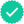#### Related questions

Question
100%

The equation is the integral of (x^3)(sqrt(1+x^2). The steps say that u=(1/2)x^2, where do they get that from?

Expert SolutionKnowledge Booster••